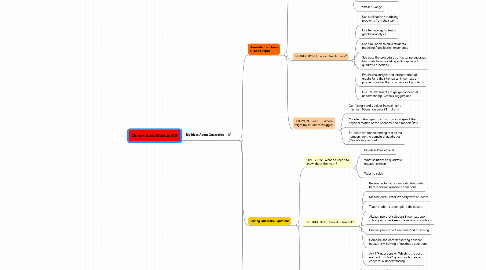Get Started. It's FreeQuadratics Startup Kit### 1.1. Quadratic Functions & Their Graphs

1.1.1. OVERVIEW: What do I need to know about the math?

1.1.1.1. Big Ideas (See emails)

1.1.1.2. Key Elements of Quadratic Graphs

1.1.1.3. Quadratic Models of Real-Life Situations

1.1.1.4. Different Forms of Quadratic Functions

1.1.1.5. Translating Between Forms

1.1.1.6. Domain & Range

1.1.2. INSTRUCTION: How do I teach these?

1.1.2.1. Use application / modeling problems from the start

1.1.2.2. Use technology to teach graphical analysis

1.1.2.3. Use simulation to build students' modeling / application experience

1.1.2.4. Separate the procedures of factoring quadratic trinomials from modeling and graphing of quadratic functions

1.1.2.5. Emphasize analysis and interpretation of graphs (and their key parts) in context of problems, rather than in absence of problems.

1.1.2.6. Use T/F statements to gauge conceptual understanding (Grace's suggestion)

1.1.3. COMMON ERRORS: Where might my students struggle?

1.1.3.1. Confusing x and y values in maximum / minimum (Common Error #1 in draft)

1.1.3.2. Misinterpreting graph of function as shape of the physical motion of the phenomenon it models (#5)

1.1.3.3. Students not understanding that all real numbers are the domain of quadratics (Grace's suggestion)

1.2.1. OVERVIEW: What do I need to know about the math?

1.2.1.1. Big ideas (See emails)

1.2.1.2. What solutions to quadratic equations mean

1.2.1.3. Ways to solve

1.2.2. INSTRUCTION: How do I teach it?

1.2.2.2. Discuss Zero Factor Property with students

1.2.2.3. Teach students to complete the square

1.2.2.4. Always interpret solutions in context upon solving and check reasonableness of solutions

1.2.2.5. Discuss pro-cons of each method of solving

1.2.2.6. Compare and contrast solving of linear equations v. solving of quadratic equations

1.2.2.7. Ask T/F questions or 'Which is the best method to solve?' questions to gauge conceptual understanding

1.2.3. COMMON ERRORS: Where might my students struggle?

1.2.3.1. Solving before putting equation in standard form (#2 and #4)

1.2.3.2. Extracting the values of solutions from the factored form without regard to signs and what they mean (#3)